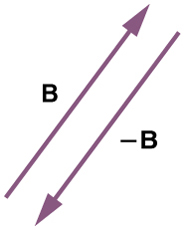# 3.2 Vector addition and subtraction: graphical methods  (Page 3/15)

 Page 3 / 15

Here, we see that when the same vectors are added in a different order, the result is the same. This characteristic is true in every case and is an important characteristic of vectors. Vector addition is commutative    . Vectors can be added in any order.

$\mathbf{\text{A}}+\mathbf{\text{B}}=\mathbf{\text{B}}+\mathbf{\text{A}}\text{.}$

(This is true for the addition of ordinary numbers as well—you get the same result whether you add $\mathbf{\text{2}}+\mathbf{\text{3}}$ or $\mathbf{\text{3}}+\mathbf{\text{2}}$ , for example).

## Vector subtraction

Vector subtraction is a straightforward extension of vector addition. To define subtraction (say we want to subtract $\mathbf{\text{B}}$ from $\mathbf{\text{A}}$ , written $\mathbf{\text{A}}–\mathbf{\text{B}}$ , we must first define what we mean by subtraction. The negative of a vector $\mathbf{\text{B}}$ is defined to be $\mathbf{\text{–B}}$ ; that is, graphically the negative of any vector has the same magnitude but the opposite direction , as shown in [link] . In other words, $\mathbf{\text{B}}$ has the same length as $\mathbf{\text{–B}}$ , but points in the opposite direction. Essentially, we just flip the vector so it points in the opposite direction.The negative of a vector is just another vector of the same magnitude but pointing in the opposite direction. So B size 12{B} {} is the negative of –B size 12{ ital "-B"} {} ; it has the same length but opposite direction.

The subtraction of vector $\mathbf{\text{B}}$ from vector $\mathbf{\text{A}}$ is then simply defined to be the addition of $\mathbf{\text{–B}}$ to $\mathbf{\text{A}}$ . Note that vector subtraction is the addition of a negative vector. The order of subtraction does not affect the results.

This is analogous to the subtraction of scalars (where, for example, ). Again, the result is independent of the order in which the subtraction is made. When vectors are subtracted graphically, the techniques outlined above are used, as the following example illustrates.

## Subtracting vectors graphically: a woman sailing a boat

A woman sailing a boat at night is following directions to a dock. The instructions read to first sail 27.5 m in a direction $\text{66.0º}$ north of east from her current location, and then travel 30.0 m in a direction $\text{112º}$ north of east (or $\text{22.0º}$ west of north). If the woman makes a mistake and travels in the opposite direction for the second leg of the trip, where will she end up? Compare this location with the location of the dock.

Strategy

We can represent the first leg of the trip with a vector $\mathbf{\text{A}}$ , and the second leg of the trip with a vector $\mathbf{\text{B}}$ . The dock is located at a location $\mathbf{\text{A}}+\mathbf{\text{B}}$ . If the woman mistakenly travels in the opposite direction for the second leg of the journey, she will travel a distance $B$ (30.0 m) in the direction $180º–112º=68º$ south of east. We represent this as $\mathbf{\text{–B}}$ , as shown below. The vector $\mathbf{\text{–B}}$ has the same magnitude as $\mathbf{\text{B}}$ but is in the opposite direction. Thus, she will end up at a location $\mathbf{\text{A}}+\left(\mathbf{\text{–B}}\right)$ , or $\mathbf{\text{A}}–\mathbf{\text{B}}$ .

We will perform vector addition to compare the location of the dock, , with the location at which the woman mistakenly arrives, .

Solution

(1) To determine the location at which the woman arrives by accident, draw vectors $\mathbf{\text{A}}$ and $\mathbf{\text{–B}}$ .

(2) Place the vectors head to tail.

(3) Draw the resultant vector $\mathbf{R}$ .

(4) Use a ruler and protractor to measure the magnitude and direction of $\mathbf{R}$ .

In this case, $R=\text{23}\text{.}\text{0 m}$ and $\theta =7\text{.}\text{5º}$ south of east.

(5) To determine the location of the dock, we repeat this method to add vectors $\mathbf{\text{A}}$ and $\mathbf{\text{B}}$ . We obtain the resultant vector $\mathbf{\text{R}}\text{'}$ :

In this case and  north of east.

We can see that the woman will end up a significant distance from the dock if she travels in the opposite direction for the second leg of the trip.

Discussion

Because subtraction of a vector is the same as addition of a vector with the opposite direction, the graphical method of subtracting vectors works the same as for addition.

does the force in a system result in the energy transfer?
full meaning of GPS system
how to prove that Newton's law of universal gravitation F = GmM ______ R²
sir dose it apply to the human system
prove that the centrimental force Fc= M1V² _________ r
prove that centripetal force Fc = MV² ______ r
Kaka
how lesers can transmit information
griffts bridge derivative
below me
please explain; when a glass rod is rubbed with silk, it becomes positive and the silk becomes negative- yet both attracts dust. does dust have third types of charge that is attracted to both positive and negative
what is a conductor
Timothy
hello
Timothy
below me
why below you
Timothy
no....I said below me ...... nothing below .....ok?
dust particles contains both positive and negative charge particles
Mbutene
corona charge can verify
Stephen
when pressure increases the temperature remain what?
remains the temperature
betuel
what is frequency
define precision briefly
CT scanners do not detect details smaller than about 0.5 mm. Is this limitation due to the wavelength of x rays? Explain.
hope this helps
what's critical angle
The Critical Angle Derivation So the critical angle is defined as the angle of incidence that provides an angle of refraction of 90-degrees. Make particular note that the critical angle is an angle of incidence value. For the water-air boundary, the critical angle is 48.6-degrees.
okay whatever
Chidalu
pls who can give the definition of relative density?
Temiloluwa
the ratio of the density of a substance to the density of a standard, usually water for a liquid or solid, and air for a gas.
Chidalu
What is momentum
mass ×velocity
Chidalu
it is the product of mass ×velocity of an object
Chidalu
how do I highlight a sentence]p? I select the sentence but get options like copy or web search but no highlight. tks. src
then you can edit your work anyway you wantByByBy Dionne MahaffeyBy Michael PittByBy Hannah ShethBy OpenStaxBy Alec MoffitBy Brenna FikeBy Richley CrapoBy OpenStaxBy